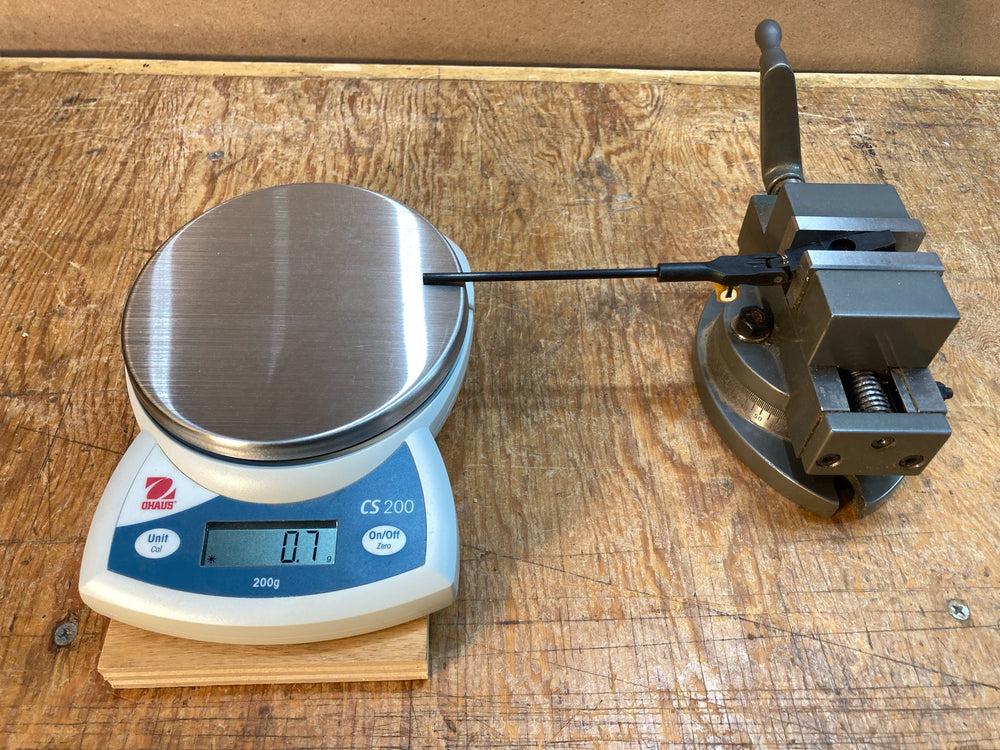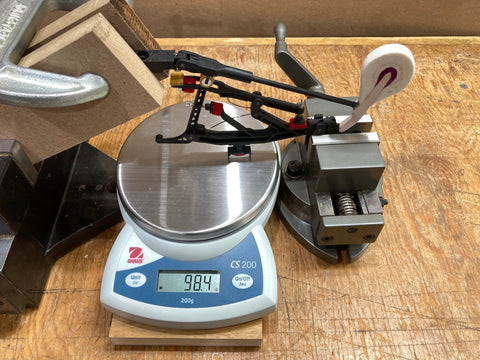# WEIGHING OFF THE GRAND ACTION – Part 7

### Playing with Proportions:

How do we become professionally conversant with the things we think we know? Experience will prove or disprove many details but without a concerted effort, these are hard to consolidate into an overall and useful understanding. Over and over, I have observed the action tradeoff between distance and weight, but how clear am I on the full implications and applications of this relationship? A simple model might help.

Using measurements from an action in my shop, let’s go through creating and and then testing a formula, not to generate precise answers so much as to understand and anticipate what will happen when proportions in the system are changed. I am not a mathematician, but as a piano technician, I regularly work with logic. If my terms vary from a norm, please forgive me (or correct me).

The hammer shank, whippen, and key act as simple levers, each with effort arm, fulcrum, and resistance arm. By measuring and weighing some parts, we can first compute and then confirm their setup. The hammer is lifted by the shank leverage, which is lifted by the whippen leverage, which is lifted by the key leverage. Although the resistance forces work vertically in relation to gravity and are changing as they travel in their arcs from rest to strike, proportions from their geometry will serve our need to anticipate outcomes. And we only have to add in the weight of the upper two levers for the numbers to work.

For samples, let’s choose two low bass hammers, a natural and a sharp. They are in the section most challenged by friction and weight and have the diagnostic benefit of a fixed default upweight. 20 grams is for me both a firm minimum, below which repetition is in jeopardy, and a firm maximum, above which the action’s heaviest hammers feel heavier than necessary.

Our sample hammers weigh 8.9 grams, with a bore distance of 2.0” and a hanging distance of 5.25” out shanks that each have an on-the-rail weight of 0.7 grams. The shanks have an 18mm (.71”) knuckle-to-center distance and the knuckles are 13mm (.51”) tall (from center pin height in the horizontal shank to contact surface of the knuckle). The math for this model will be computed in grams for weight/friction and inches for distance.The hammer/shank lever arms are each the hypotenuse of their assembly specs. We can solve for them using the Pythagorean theorem a² + b² = c², where a = side of right triangle (bore distance), b = side of right triangle (hang distance), and c = the hypotenuse (length of resistance arm). An online calculator makes the computations easy, but these distances can also be measured directly from the parts. The shank effort arm extends from contact surface of knuckle to flange center and its resistance arm goes from flange center to crown of hammer.

Shank Leverage (SL):
RA (resistance arm) = √ (bore distance² + hang distance²)
RA = √ (2.02 + 5.252) = 5.62
EA (effort arm) = √ (knuckle distance² + knuckle height²)
EA = √ (.712 + .512) = .87
SL = RA ÷ EA

SL = 5.62 ÷ .87 = 6.46

So, the shank leverage of 6.46 times the hammer weight of 8.9 grams plus the shank weight of .7 grams will add up to the hammer resistance weight (HR) that bears on the jack/rep lever surfaces of the whippen.

Hammer resistance weight (HR):
HR = SL • HW (hammer weight) + SW (shank weight)

HR = 6.46 • 8.9 + .7 = 58.2 grams

The whippen’s effort arm is from where heel contacts capstan to flange center (natural 2.61, sharp 2.64 since sharp heels are 2mm taller than natural heels) and the resistance arm is from flange center to mid jack tip (3.75).

Whippen leverage (WL):
WL = RA ÷ EA
Natural WL = 3.75 ÷ 2.61 = 1.44

Sharp WL = 3.75 ÷ 2.64 = 1.42

The whippen resistance weight is calculated from the whippen leverage times the hammer resistance weight plus the whippen weight (natural 14.6 grams weighed at the heel when attached to its rail, sharp 14.7 because of its taller heel).

Whippen resistance weight (WR):
WR = WL • HR + WW (whippen weight)
Natural WR =  1.44 • 58.2 + 14.6 = 98.4

Sharp WR = 1.42 • 58.2 + 14.7 = 97.3

The key’s effort arm is from keytop over front keypin to underside of key mid balance hole (natural 8.5, sharp 6.75) and its resistance arm is from balance hole to mid capstan surface (natural 4.5, sharp 3.95).Key leverage (KL):
KL = RA ÷ EA
Natural KL = 4.5 ÷ 8.5 = .53

Sharp KL = 3.95 ÷ 6.75 = .59

And the key’s downweight is computed by key leverage times WR (which includes HR):

Downweight (DW):
DW = WR • KL
Natural DW = 98.4 • .53 = 52

Sharp DW = 97.3 • .59 = 57

The key is weighed off so that, loaded at the front with the 20-gram minimum upweight, the key, when released from full dip, returns its hammer entirely to rest at the same speed as the DW raises the hammer to letoff. This is a liberally interpreted, fast moving upweight – If you tap the topstack, the same key will lift 25 grams slowly tap by tap.

Upweight (UW):

UW = 20

Halfway between DW and UW is a weight that, if placed at the front of the key, will balance the key when neither end touches keyframe. Weight added to the key on either side of the balancerail will then favor moving the key down in that direction and will do that if it can overcome the system's friction. DW will depress the key but only to where letoff and drop engage the whippen. UW on the depressed front of the key will be lifted so the key goes down at the back and returns to rest.

Balanceweight (BW):
BW = (DW + UW) ÷ 2
Natural BW = (52 + 20) ÷ 2 = 36

Sharp BW = (57 + 20) ÷ 2 = 38.5

The difference between BW and DW or BW and UW is split between friction at the fulcrums and contact friction between parts.

Friction (F):
F = (DW – UW) ÷ 2
Natural F = (52 – 20) ÷ 2 = 16

Sharp F = (57 – 20) ÷ 2 = 18.5

Key leverage, however, depends on where a finger plays the key, and it changes as the point of measurement moves back on the keytop. The natural that weighs off at 52/20 at the front of the key (BW 36), changes to 69/27 (BW 48) halfway back, and to 114/44 (BW 79) at the back. For sharps, weighoff changes from 57/20 (BW 38.5) at the front to 69/25 (BW 47) in the middle and 90/32 (BW 61) at the back.

And friction rises proportionally with the rising weight front-of-key to back-of-key: natural 16 to 21 to 35, sharp 18.5 to 22 to 31. Note how similar weight and friction are in natural and sharp in the middle area where fingers play them side-by-side.A final set of numbers reviews how the three contiguous leverages combine with lever weights to anticipate the effects of hammer, shank, whippen, key, and friction choices. This is the model:

Downweight from hammer weight:
DW = WR • KL
DW = ((HW • SL + SW) • WL + WW) • KL
BW = (DW + UW) ÷ 2
F = (DW – UW) ÷ 2
Natural: DW = ((8.9 • 6.46 + .7) • 1.44 + 14.6) • .53  = 52
UW = 20
BW = (52 + 20) ÷ 2 = 36
F = (52 – 20) ÷ 2 = 16
Sharp: DW = ((8.9 • 6.46 + .7) • 1.42 + 14.6) • .59  = 57
UW = 20
BW = (57 + 20) ÷ 2 = 38.5

F = (52 – 20) ÷ 2 =  18.5

These computations require the following:
• Hammer resistance arm distance
• Hammer effort arm distance
• Hammer weight
• Hammer shank weight
• Whippen resistance arm distance
• Whippen effort arm distance
• Whippen weight
• Key resistance arm distance
• Key effort arm distance
• Fully regulating sample natural and sample sharp
• Weighing off samples with weight (lead or copper) on key to confirm UW/DW
• Testing/adjusting friction points as needed

By estimating changes and running them through the model, we can get a sense of what will happen and in what proportion compared to other changed or not-changed items. The last three items on this list are key to proving the validity of what we have decided to do or not do and should be included in any full regulation as early in the game as possible. The sampling takes a little time but pays off when the 88 executions of the plan for prep and regulating are right on target compared with something going wrong and having to re-execute one or more steps times 88.

Let’s try out the model, now, to see what happens with a change of hammer weight. Because leverage in the shank is so much greater than in whippen or key, it has way more influence on balance and playability. Let’s start by increasing HW by one gram in the natural sample:

HW = 8.9 + 1.0 = 9.9
DW = ((9.9 • 6.46 + .7) • 1.44 + 14.6) • .53  = 57
UW = 25
BW = (57 + 25) ÷ 2 = 41

F = (57 – 25) ÷ 2 = 16

Adding 1 gram of HW, then, raises DW by 5 grams. This also raises BW by 5 grams since it would take the addition of the same weight on the other side of the balancerail to rebalance. Friction may rise an amount proportional to the weight increase, but it must be a small proportion as testing shows no change in friction. DW, UW, and BW, however, all change the same amount in the same direction. In lowering HW 1 gram, the same thing happens. DW, UW, and BW all drop 5 grams with no detectable change in F:

HW = 8.9 - 1.0 = 7.9
DW = ((7.9 • 6.46 + .7) • 1.44 + 14.6) • .53  = 47
UW = 15
BW = (47 + 15) ÷ 2 = 31

F = (47 – 15) ÷ 2 = 16

Extra hammer weight may have tonal benefits but can add the cost of weighing off to lower DW. And it increases inertia in the system (more on that next month). But lowering the HW would require a weighoff to make the UW acceptable. And this process would mean removing lead, drilling out holes, plugging them, and weighing off. But there would be two desirable affects: lowering inertia with less hammer weight (times the leverages) and further lowering it with less compensatory lead in the keys .

• Part 1 "Why Weigh Off?"
• Part 2 "My Strategy"
• Part 3 "Method and Style"
• Part 3a "Regulation Strategy Review"
• Part 4 "Pragmatics"
• Part 5 "Trials and Errors"
• Part 6 "Levers"
• Part 8 "Weight, Friction, Inertia, and Distance"
• Part 9 "Plan by Number?"
• Part 10 "Best Balance for Best Art"

•

### Be the first to comment

All comments are moderated before being published